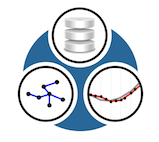EPANET-RTX  1.1 real-time extension for the EPANET toolkit
Config File Comparison

# Forward Simulation Configuration File

# sampletown configuration file -- forward simulation of sampletown network
version = "1.0";
configuration:
{
# point records
records = (
{
# Specify a MySQL database.
# Uses the MySQL C++ Connector, based on the JDBC API.
# or "unix://path/to/unix_socket_file"
name = "sampletown_synthetic";
type = "MySQL";
connection = "DB=RTX_sampletown_synthetic;UID=rtx_db_agent;PWD=rtx_db_agent;HOST=tcp://localhost;";
}
); // records
simulation = {
# specify hyd and wq timesteps - this overrides whatever is in the model file
time = {
hydraulic = 60;
quality = 60;
};
}; // simulation
save = {
# staterecord - specify the name of a record (from above section)
# this will store all hydraulic state information (head, flow, demand)
staterecord = "sampletown_synthetic";
save_states = ( "all" );
};
model = {
file = "sampletown.inp";
# type -- either "synthetic_epanet" (model-based control rules) or "epanet" (control rules are stripped)
type = "synthetic_epanet";
}; // model
}; // configuration

# Real-Time Simulation Configuration File

# sampletown configuration file - realtime version
version = "1.0";
configuration:
{
# point records
records = (
{
name = "sampletown_synthetic";
type = "MySQL";
connection = "DB=RTX_sampletown_synthetic;UID=rtx_db_agent;PWD=rtx_db_agent;HOST=tcp://localhost;";
},
{
name = "sampletown_realtime";
type = "MySQL";
connection = "DB=RTX_sampletown_realtime;UID=rtx_db_agent;PWD=rtx_db_agent;HOST=tcp://localhost;";
}
); // records
# regular clocks
clocks = (
{
name = "1m";
type = "regular";
period = 60;
start = 0;
},
{
name = "10m";
type = "regular";
period = 600; // == 10 minutes
start = 0;
}
); // clocks
timeseries = (
# source time series - these are not strictly regular
{
name = "N Mills demand";
type = "TimeSeries";
description = "Mills demand - metered";
pointRecord = "sampletown_synthetic";
units = "gpm";
},
{
name = "L ReservoirCheckValve flow";
type = "TimeSeries";
description = "supply flow";
pointRecord = "sampletown_synthetic";
units = "gpm";
},
{
type = "TimeSeries";
pointRecord = "sampletown_synthetic";
units = "ft";
},
{
name = "L 4 flow";
type = "TimeSeries";
description = "interzone flow meter";
pointRecord = "sampletown_synthetic";
units = "gpm";
},
# resamplers - so that we have regular time series
{
name = "Mills Demand (resampled)";
type = "Resampler";
clock = "1m";
source = "N Mills demand";
units = "gpm";
},
{
name = "Supply flow (resampled)";
type = "Resampler";
clock = "1m";
source = "L ReservoirCheckValve flow";
units = "gpm";
},
{
type = "Resampler";
clock = "1m";
units = "ft";
},
{
name = "Interzone Flow (resampled)";
type = "Resampler";
clock = "1m";
source = "L 4 flow";
units = "gpm";
},
// water quality signals
{
name = "node quality";
type = "Constant";
clock = "1m";
units = "mg/l";
value = 12;
}
); // end timeseries
model = {
file = "sampletown.inp";
type = "epanet";
}; // model
elements = (
# associate source data with model elements (and define which parameter they are linked to)
# metered demand
{
model_id = "Mills";
timeseries = "Mills Demand (resampled)";
parameter = "flow_boundary";
},
# tank
{
model_id = "NewportTank";
},
# supply
{
model_id = "ReservoirCheckValve";
timeseries = "Supply flow (resampled)";
parameter = "flow_measure";
},
# flow
{
model_id = "4";
timeseries = "Interzone Flow (resampled)";
parameter = "flow_measure";
},
# water quality
{
model_id = "TreatmentPlant";
timeseries = "node quality";
parameter = "quality_boundary";
}
); // elements
# simulation properties
simulation = {
time = {
hydraulic = 600; // 10m
quality = 60; // 1m
};
}; // simulation
save = {
staterecord = "sampletown_realtime";
// save_states = ( "measured", "dma_demand" );
save_states = ( "all" );
/* states == all / head / flow / quality / energy / demand / dma_demand / status / setting / error / measured*/
};
dma = {
auto_detect = true;